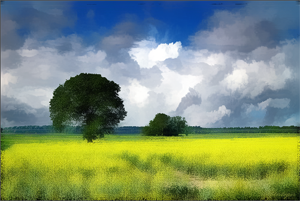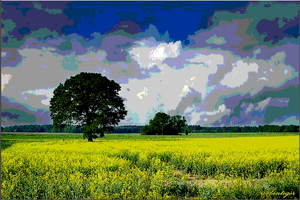Everyone already knows about the new Flash player 10 (Astro) features that adobe presented at MAX, and above all the new image processing language (Hydra) that it will support in the future.

Today I finally found a couple of hours to play with AIF, even if no more because of my limitations knowledge of image processing algorithms 🙂
In fact I was able to broke different times my video card driver (with a panic black screen)!

Anyway after all I did just a couple of experiments trying to convert a couple of scripts I already did using the old flash 9 bitmapdata filters..```kernel Snn { parameter float size < minValue: 0.0; maxValue: 8.0; defaultValue: 2.0; >; parameter int step < minValue: 1; maxValue: 10; defaultValue: 1; >; void evaluatePixel(in image4 src, out pixel4 result) { pixel2 coord = outCoord(); pixel4 valueC = sampleLinear(src, coord); float theStep = float(step); float2 point = float2(0.0,0.0); float x = 0.0; float y = 0.0; float count = 0.0; pixel4 sum = pixel4(0.0, 0.0, 0.0, 0.0); pixel4 valueA; pixel4 valueB; for( x = - size; x < size; x+= theStep) { for(y = -size; y < size; y+= theStep) { valueA = sampleLinear(src, pixel2(coord.x + x, coord.y + y)); valueB = sampleLinear(src, pixel2(coord.x - x, coord.y - y)); if ( any(lessThan(abs(valueC - valueA), abs(valueC - valueB)))) { sum = sum + valueA; } else { sum = sum + valueB; } count = count + 1.0; } } result = sum/count; } }``````kernel Websafe { parameter float value < minValue: 0.0; maxValue: 500.0; defaultValue: 10.0; >; void evaluatePixel( in image4 src, out pixel4 result) { result = sampleLinear(src, outCoord()); result = (floor((result*255.0)/51.0) * 51.0)/255.0; } }```
As you can see it’s nothing new, they’re just my first steps in this new language.. don’t spend much time on these example, look at the coolest examples shown in the Hydra gallery instead!

Share with...